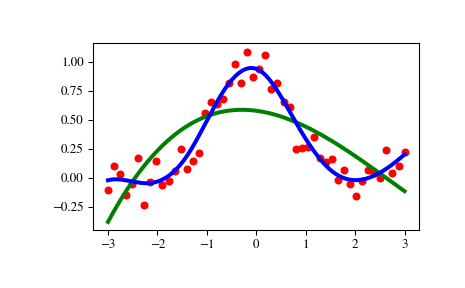# scipy.interpolate.UnivariateSpline¶

class scipy.interpolate.UnivariateSpline(x, y, w=None, bbox=[None, None], k=3, s=None, ext=0, check_finite=False)[source]

One-dimensional smoothing spline fit to a given set of data points.

Fits a spline y = spl(x) of degree k to the provided x, y data. s specifies the number of knots by specifying a smoothing condition.

Parameters: x : (N,) array_like 1-D array of independent input data. Must be increasing. y : (N,) array_like 1-D array of dependent input data, of the same length as x. w : (N,) array_like, optional Weights for spline fitting. Must be positive. If None (default), weights are all equal. bbox : (2,) array_like, optional 2-sequence specifying the boundary of the approximation interval. If None (default), bbox=[x, x[-1]]. k : int, optional Degree of the smoothing spline. Must be <= 5. Default is k=3, a cubic spline. s : float or None, optional Positive smoothing factor used to choose the number of knots. Number of knots will be increased until the smoothing condition is satisfied: sum((w[i] * (y[i]-spl(x[i])))**2, axis=0) <= s  If None (default), s = len(w) which should be a good value if 1/w[i] is an estimate of the standard deviation of y[i]. If 0, spline will interpolate through all data points. ext : int or str, optional Controls the extrapolation mode for elements not in the interval defined by the knot sequence. if ext=0 or ‘extrapolate’, return the extrapolated value. if ext=1 or ‘zeros’, return 0 if ext=2 or ‘raise’, raise a ValueError if ext=3 of ‘const’, return the boundary value. The default value is 0. check_finite : bool, optional Whether to check that the input arrays contain only finite numbers. Disabling may give a performance gain, but may result in problems (crashes, non-termination or non-sensical results) if the inputs do contain infinities or NaNs. Default is False.

InterpolatedUnivariateSpline
Subclass with smoothing forced to 0
LSQUnivariateSpline
Subclass in which knots are user-selected instead of being set by smoothing condition
splrep
An older, non object-oriented wrapping of FITPACK
BivariateSpline
A similar class for two-dimensional spline interpolation

Notes

The number of data points must be larger than the spline degree k.

NaN handling: If the input arrays contain nan values, the result is not useful, since the underlying spline fitting routines cannot deal with nan . A workaround is to use zero weights for not-a-number data points:

>>> from scipy.interpolate import UnivariateSpline
>>> x, y = np.array([1, 2, 3, 4]), np.array([1, np.nan, 3, 4])
>>> w = np.isnan(y)
>>> y[w] = 0.
>>> spl = UnivariateSpline(x, y, w=~w)


Notice the need to replace a nan by a numerical value (precise value does not matter as long as the corresponding weight is zero.)

Examples

>>> import matplotlib.pyplot as plt
>>> from scipy.interpolate import UnivariateSpline
>>> x = np.linspace(-3, 3, 50)
>>> y = np.exp(-x**2) + 0.1 * np.random.randn(50)
>>> plt.plot(x, y, 'ro', ms=5)


Use the default value for the smoothing parameter:

>>> spl = UnivariateSpline(x, y)
>>> xs = np.linspace(-3, 3, 1000)
>>> plt.plot(xs, spl(xs), 'g', lw=3)


Manually change the amount of smoothing:

>>> spl.set_smoothing_factor(0.5)
>>> plt.plot(xs, spl(xs), 'b', lw=3)
>>> plt.show()Methods

 __call__(x[, nu, ext]) Evaluate spline (or its nu-th derivative) at positions x. antiderivative([n]) Construct a new spline representing the antiderivative of this spline. derivative([n]) Construct a new spline representing the derivative of this spline. derivatives(x) Return all derivatives of the spline at the point x. get_coeffs() Return spline coefficients. get_knots() Return positions of interior knots of the spline. get_residual() Return weighted sum of squared residuals of the spline approximation. integral(a, b) Return definite integral of the spline between two given points. roots() Return the zeros of the spline. set_smoothing_factor(s) Continue spline computation with the given smoothing factor s and with the knots found at the last call.